Case Based Questions (MCQ)

Chapter 3 Class 12 Matrices
Serial order wise

## Amit, Biraj and Chirag were given the task of creating a square matrix of order 2. Below are the matrices created by them. A, B , C are the matrices created by Amit, Biraj and Chirag respectively. A = [1 2 -1 3]   B = [4 0 1 5]  C = [2 0 1 -2] If a = 4 and b = −2, based on the above information answer the following: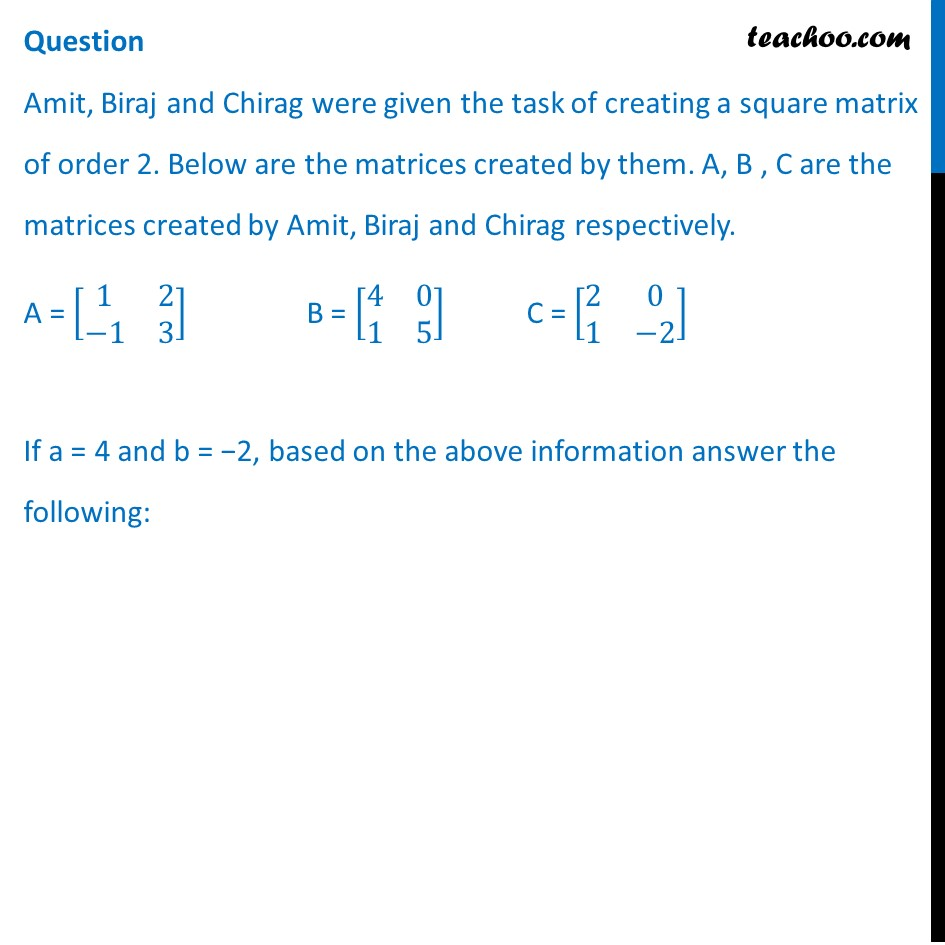## (a) [1 6       2 7] (b) [6 1       7 2] (c) [7 2       1 6] (d) [2 1       7 6]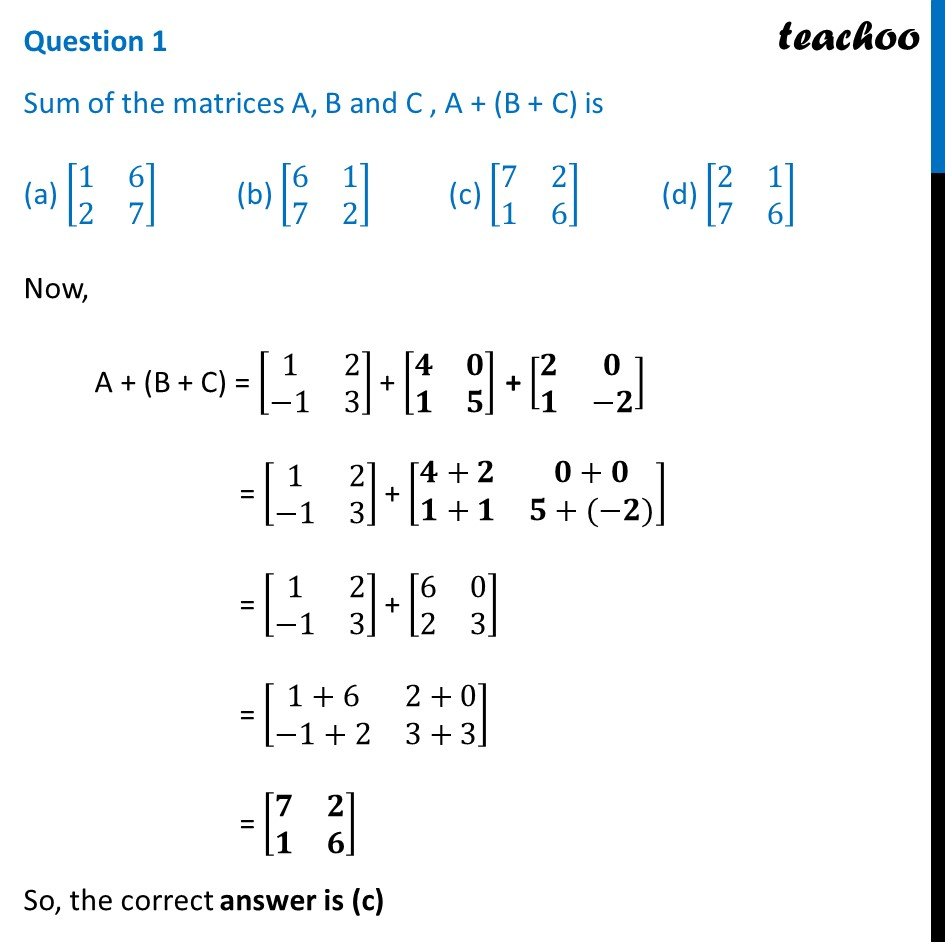## (a)  [1 2       -1 3] (b)  [2 1       3 -1] (c)  [1 -1       2 3] (d)  [2 3       1 -1]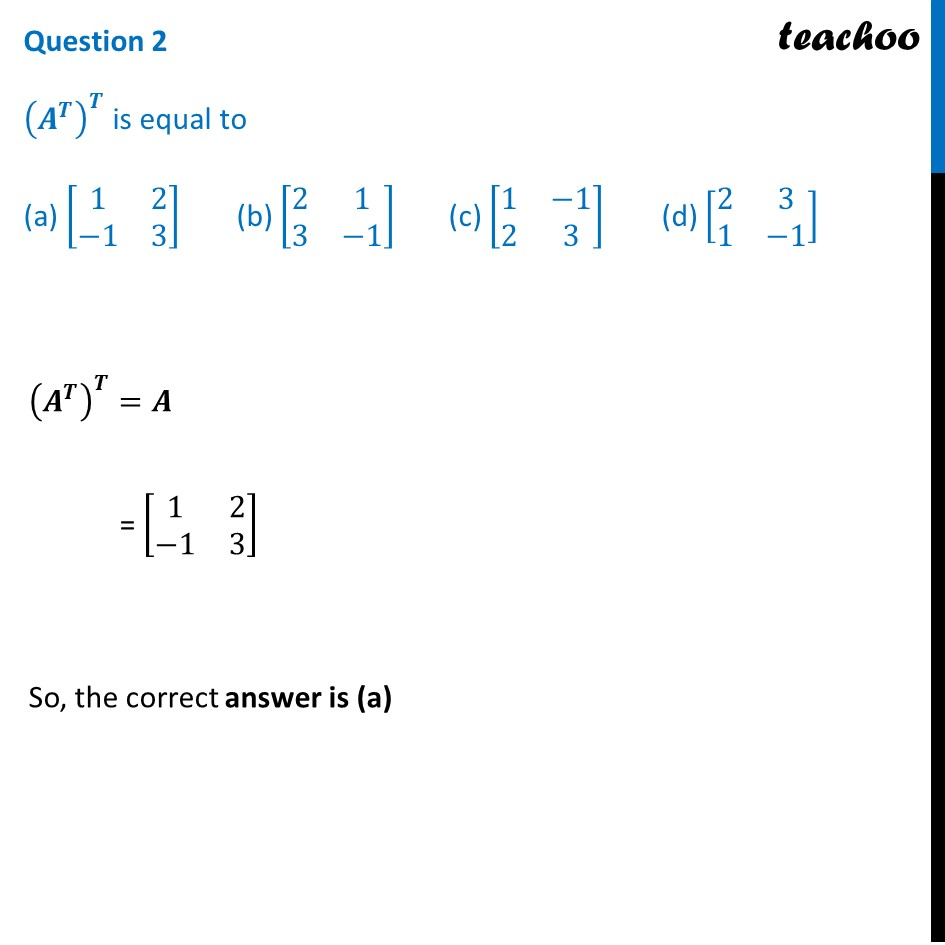## (a)  [-2  -4         2  -6] (b)   [-2  2       -4  -6] (c)  [-2  2       -6 -4] (d)  [-6  -2         2  4]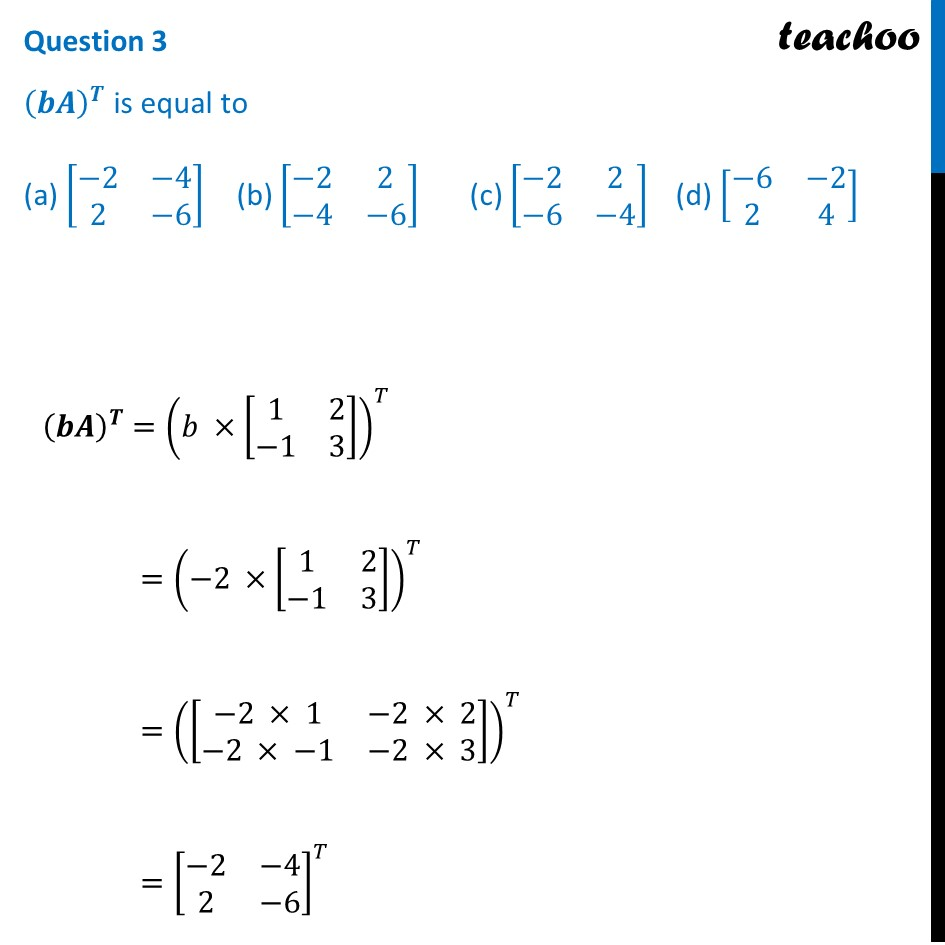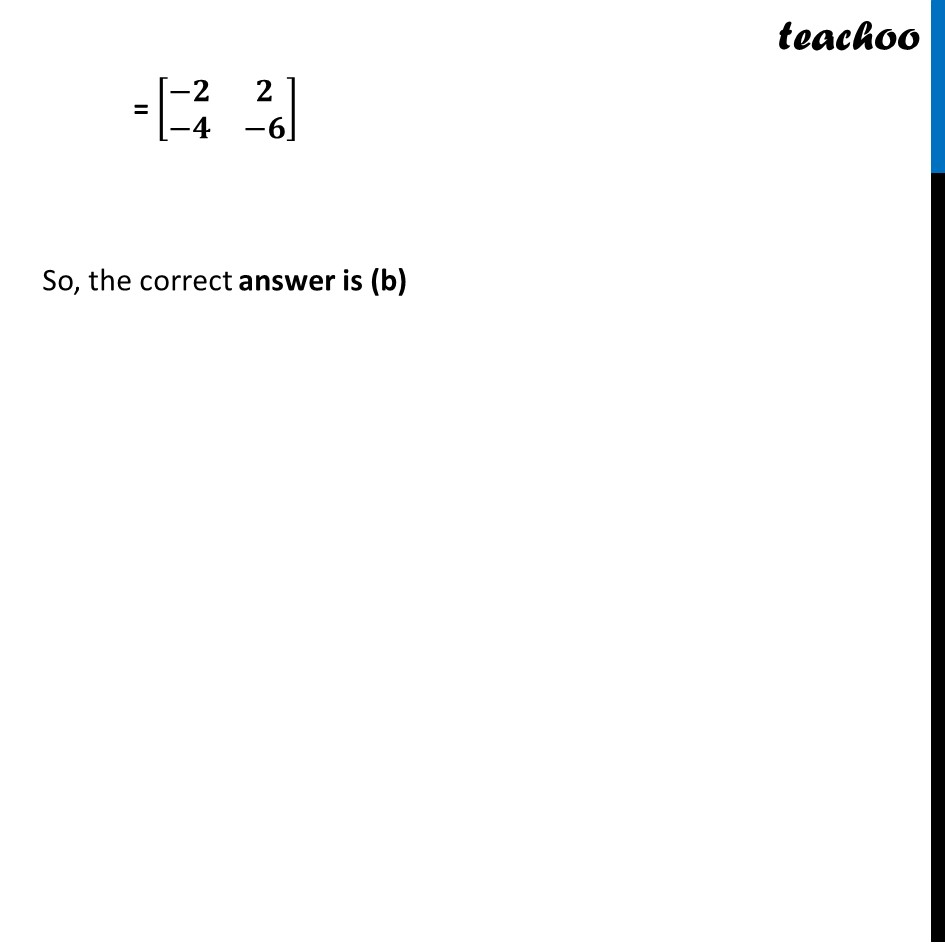## (a)  [-4  -6        -4   4] (b)  [-4  -4         4   -6] (c)  [-4  -4        -6  -4] (d)  [-6  4        -4  -4]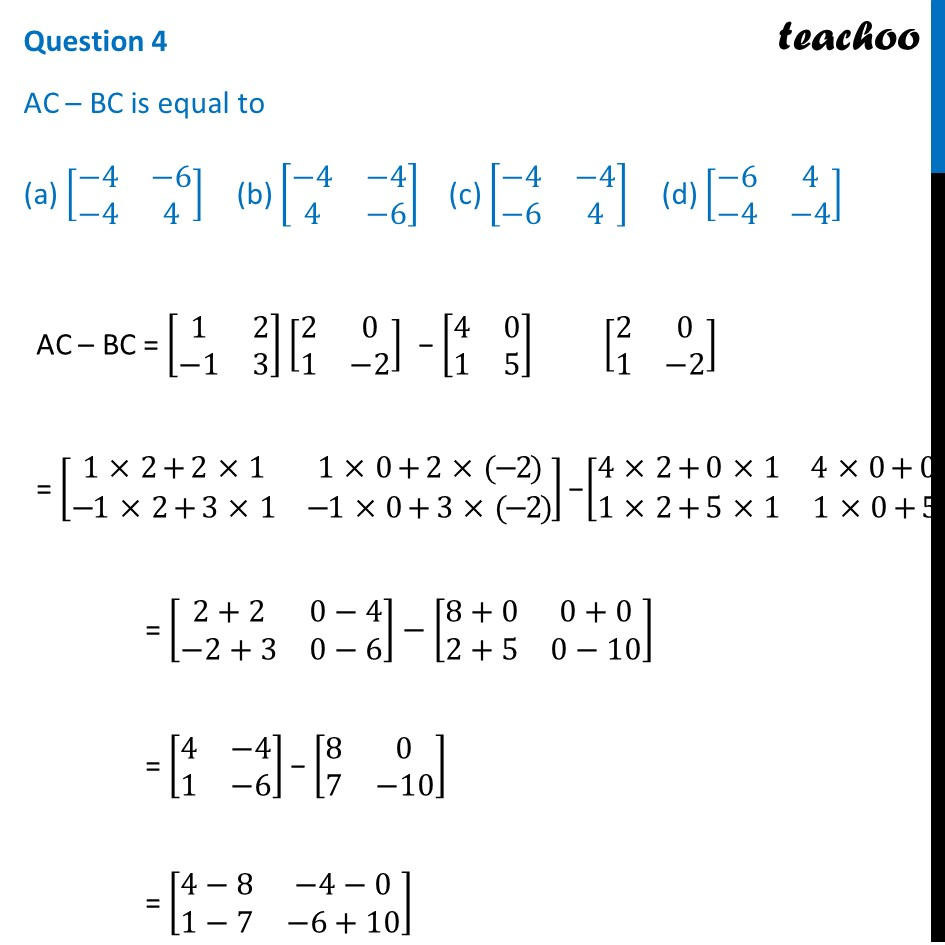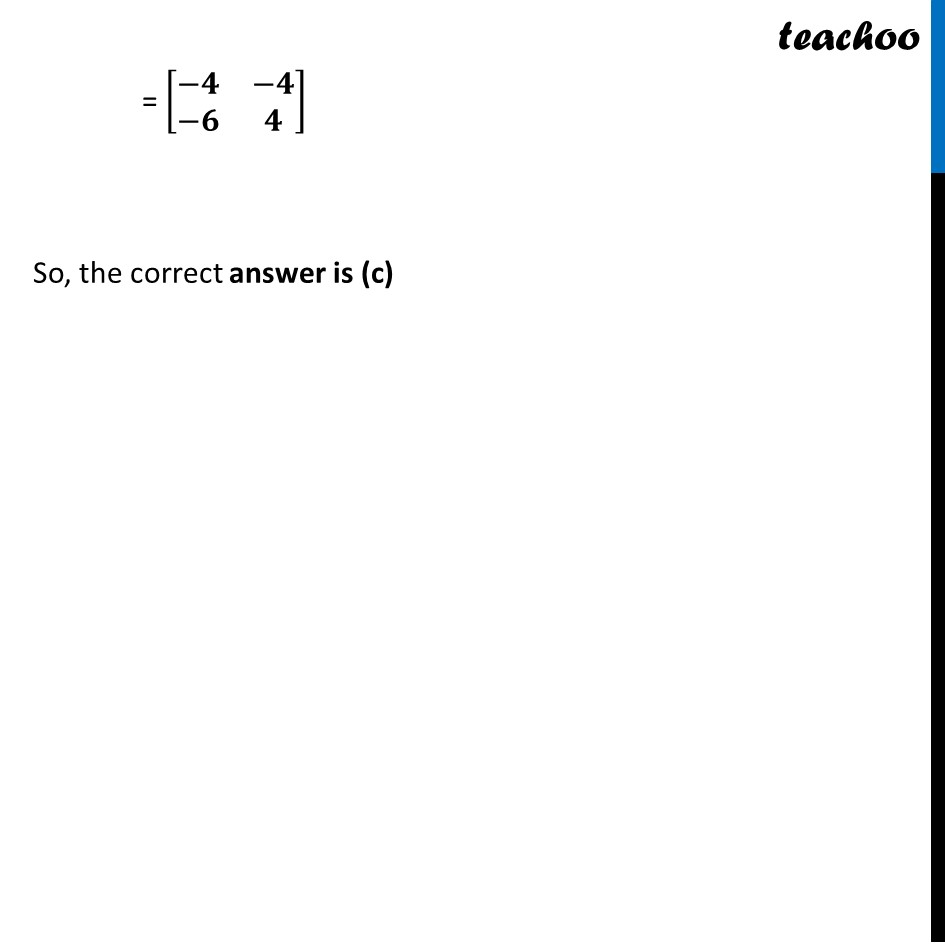## (a)  [0  8        10  2] (b)  [2  10        8  0] (c)  [8  0        2  10] (d)  [2  0        8  10]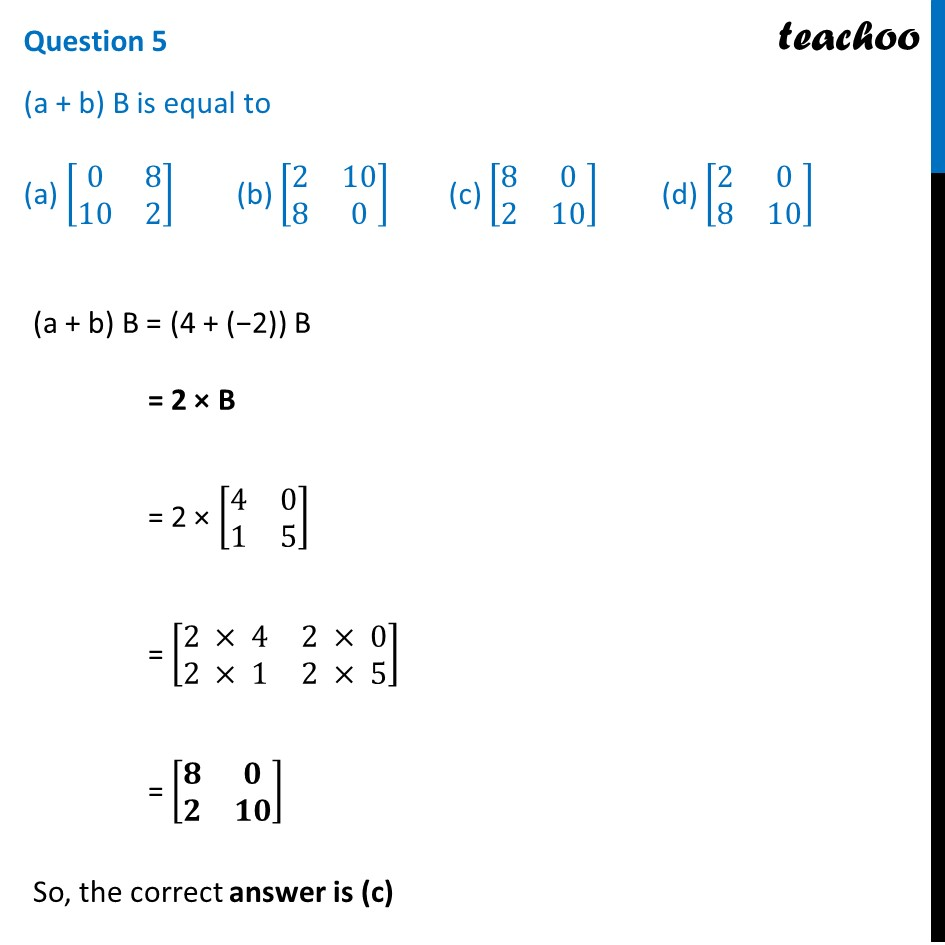Learn in your speed, with individual attention - Teachoo Maths 1-on-1 Class

### Transcript

Question Amit, Biraj and Chirag were given the task of creating a square matrix of order 2. Below are the matrices created by them. A, B , C are the matrices created by Amit, Biraj and Chirag respectively. A = [■8(1&2@−1&3)] B = [■8(4&0@1&5)] C = [■8(2&0@1&−2)] If a = 4 and b = −2, based on the above information answer the following: Question 1 Sum of the matrices A, B and C , A + (B + C) is (a) [■8(1&6@2&7)] (b) [■8(6&1@7&2)] (c) [■8(7&2@1&6)] (d) [■8(2&1@7&6)] Now, A + (B + C) = [■8(1&2@−1&3)] + [■8(𝟒&𝟎@𝟏&𝟓)] + [■8(𝟐&𝟎@𝟏&−𝟐)] = [■8(1&2@−1&3)] + [■8(𝟒+𝟐&𝟎+𝟎@𝟏+𝟏&𝟓+(−𝟐))] = [■8(1&2@−1&3)] + [■8(6&0@2&3)] = [■8(1+6&2+0@−1+2&3+3)] = [■8(𝟕&𝟐@𝟏&𝟔)] So, the correct answer is (c) Question 2 (𝑨^𝑻 )^𝑻 is equal to (a) [■8(1&2@−1&3)] (b) [■8(2&1@3&−1)] (c) [■8(1&−1@2&3)] (d) [■8(2&3@1&−1)] (𝑨^𝑻 )^𝑻=𝑨 = [■8(1&2@−1&3)] So, the correct answer is (a) Question 3 (𝒃𝑨)^𝑻 is equal to (a) [■8(−2&−4@2&−6)] (b) [■8(−2&2@−4&−6)] (c) [■8(−2&2@−6&−4)] (d) [■8(−6&−2@2&4)] (𝒃𝑨)^𝑻 =(𝑏 ×[■8(1&2@−1&3)])^𝑇 =(−2 ×[■8(1&2@−1&3)])^𝑇 =([■8(−2 × 1&−2 × 2@−2 × −1&−2 × 3)])^𝑇 =[■8(−2&−4@2&−6)]^𝑇 = [■8(−𝟐&𝟐@−𝟒&−𝟔)] So, the correct answer is (b) Question 4 AC – BC is equal to (a) [■8(−4&−6@−4&4)] (b) [■8(−4&−4@4&−6)] (c) [■8(−4&−4@−6&4)] (d) [■8(−6&4@−4&−4)] AC – BC = [■8(1&2@−1&3)] [■8(2&0@1&−2)] − [■8(4&0@1&5)] [■8(2&0@1&−2)] = [■8(1 × 2+2 × 1&1 × 0+2 × (−2)@−1 × 2+3 × 1&−1 × 0+3 × (−2))] − [■8(4 × 2+0 × 1&4 × 0+0 × (−2)@1 × 2+5 × 1&1 × 0+5× (−2))] = [■8(2+2&0−4@−2+3&0−6)]−[■8(8+0&0+0@2+5&0−10)] = [■8(4&−4@1&−6)] − [■8(8&0@7&−10)] = [■8(4−8&−4−0@1−7&−6+10)] = [■8(−𝟒&−𝟒@−𝟔&𝟒)] So, the correct answer is (c) Question 5 (a + b) B is equal to (a) [■8(0&8@10&2)] (b) [■8(2&10@8&0)] (c) [■8(8&0@2&10)] (d) [■8(2&0@8&10)] (a + b) B = (4 + (−2)) B = 2 × B = 2 × [■8(4&0@1&5)] = [■8(2 × 4&2 × 0@2 × 1&2 × 5)] = [■8(𝟖&𝟎@𝟐&𝟏𝟎)] So, the correct answer is (c)